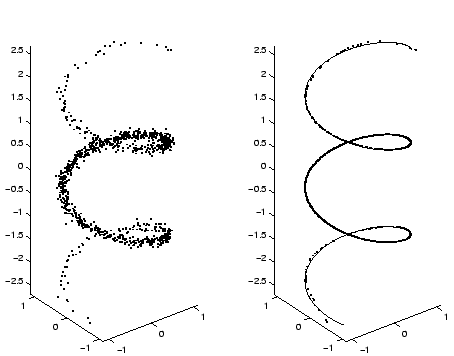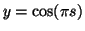Next: Nonlinear Artificial Data Up: Experiments Previous: Learning Scheme

## HelixLet us first take a look at a toy problem which shows that it is possible to find a nonlinear subspace and model it with an MLP network in an unsupervised manner. A set of 1000 data points, shown on the left plot of Fig. 6, were generated from a normally distributed source s into a helical subspace. The z-axis had a linear correspondence to the source and the x- and y-axes were sine and cosine:,and z = s. Gaussian noise with standard deviation 0.05 was added to all three data components.

One-dimensional nonlinear subspaces were estimated with the nonlinear independent factor analysis algorithm. Several different numbers of hidden neurons and initialisations of the MLP networks were tested and the network which minimised the cost function was chosen. The best network had 16 hidden neurons. The original noisy data and the means of the outputs of the best MLP network are shown in Fig. 6. It is evident that the network was able to learn the correct subspace. Only the tails of the helix are somewhat distorted. The network estimated the standard deviations of the noise on different data components to be 0.052, 0.055 and 0.050. This is in close correspondence with the actual noise level of 0.05.

This problem is not enough to demonstrate the advantages of the method since it does not prove that the method is able to deal with high dimensional latent spaces. This problem was chosen simply because it is easy to visualise.Next: Nonlinear Artificial Data Up: Experiments Previous: Learning Scheme
Harri Lappalainen
2000-03-03